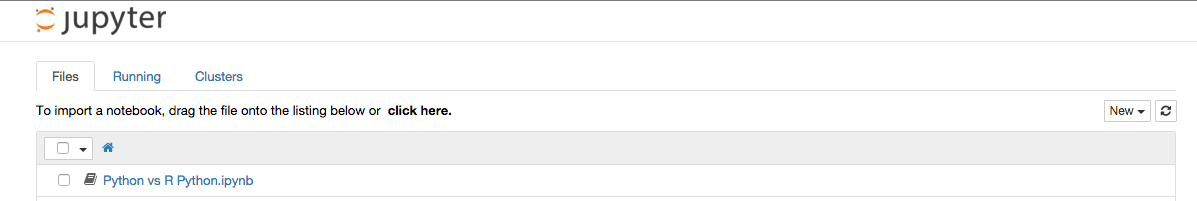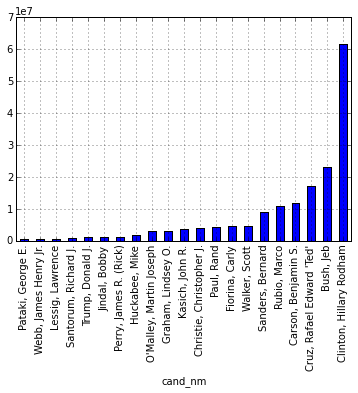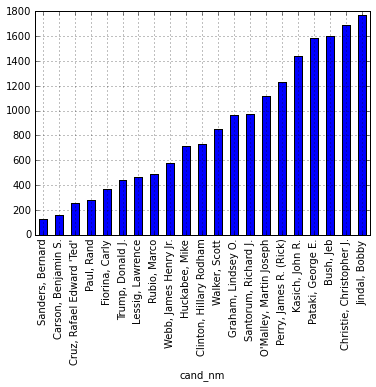# Python for data science: Getting startedPython is becoming an increasingly popular language for data science, and with good reason. It’s easy to learn, has powerful data science libraries, and integrates well with databases and tools like Hadoop and Spark. With Python, we can perform the full lifecycle of data science projects, including reading data in, analyzing data, visualizing data, and making predictions with machine learning. In this post, we’ll walk through getting started with Python for data science. If you want to dive more deeply into the topics we cover, visit Dataquest, where we teach every component of the Python data science lifecycle in depth. We’ll be working with a dataset of political contributions to candidates in the 2016 US presidential elections, which can be found here. The file is in csv format, and each row in the dataset represents a single donation to the campaign of a single candidate. The dataset has several interesting columns, including:

• `cand_nm` — name of the candidate receiving the donation.
• `contbr_nm` — name of the contributor.
• `contbr_state` — state where the contributor lives.
• `contbr_employer` — where the contributor works.
• `contbr_occupation` — the occupation of the contributor.
• `contb_receipt_amount` — the size of the contribution, in US dollars.
• `contb_receipt_dt` — the date the contribution was received.

## Installing Python

The first step we’ll need to take to analyze this data is to install

Python. Installing Python is an easy process with Anaconda, a tool that installs Python along with several popular data analysis libraries. You can download Anaconda here. It’s recommended to install Python 3.5, which is the newest version of Python. You can read more about Python 2 vs Python 3 here. Anaconda automatically installs several libraries that we’ll be using in this post, including Jupyter, Pandas, scikit-learn, and matplotlib.

## Getting started with Jupyter

Now that we have everything installed, we can launch Jupyter notebook (formerly known as IPython notebook). Jupyter notebook is a powerful data analysis tool that enables you to quickly explore data, visualize your findings, and share your results. It’s used by data scientists at organizations like Google, IBM, and Microsoft to analyze data and collaborate. Start Jupyter by running

`ipython notebook` in the terminal. If you have trouble, check here.You should see a file browser interface that allows you to create new notebooks. Create a Python 3 notebook, which we’ll be using in our analysis. If you need more help with installation, check out our guide here.

### Notebook cells

Each Jupyter notebook is composed of multiple

cells in which you can run code or write explanations. Your notebook will only have one cell initially, but you can add more:

``````
# This is a code cell.
Any output we generate here will show up below.
print(10)
b = 10
``````
``````
# You can have multiple cells, and re-run each cell as many times as you want to refine your analysis.
# The power of Jupyter notebook is that the results of each cell you run are cached.
# So you can run code in cells that depends on other cells.
print(b * 10)``````

If you want to learn more about Jupyter, check our our in-depth tutorial

here.

## Getting started with Pandas

Pandas is a data analysis library for Python. It enables us to read in data from a variety of formats, including csv, and then analyze that data efficiently. We can read in the data using this code:

``````
import pandas as pd
``donations.shape``
``(384885, 18)``
``donations.head(2)``
cmte_id cand_id cand_nm contbr_nm contbr_city contbr_st contbr_zip contbr_employer contbr_occupation contb_receipt_amt contb_receipt_dt receipt_desc memo_cd memo_text form_tp file_num tran_id election_tp
C00458844 P60006723 Rubio, Marco KIBBLE, KUMAR DPO AE 092131903 U.S. DEPARTMENT OF HOMELAND SECURITY LAW ENFORCEMENT 500 27-AUG-15 NaN NaN NaN SA17A 1029457 SA17.813360 P2016 NaN
C00458844 P60006723 Rubio, Marco HEFFERNAN, MICHAEL APO AE 090960009 INFORMATION REQUESTED PER BEST EFFORTS INFORMATION REQUESTED PER BEST EFFORTS 210 27-JUN-15 NaN NaN NaN SA17A 1029436 SA17.796904 P2016 NaN

In the cells above, we import the Pandas library using

`import pandas as pd`, then use the read_csv() method to read `political_donations.csv` into the `donations` variable. The `donations` variable is a Pandas DataFrame, which is an enhanced version of a matrix that has data analysis methods built in and allows different datatypes in each column. We access the `shape` property of `donations` variable to print out how many rows and columns it has. When a statement or variable is placed on the last line of a notebook cell, its value or output is automatically rendered! We then use the head() method on DataFrames to print out the first two rows of `donations` so we can inspect them. If you want to dive into more depth with Pandas, see our course here.

## Total donations by candidate

We can compute per-candidate summary statistics using the Pandas

groupby() method. We can first use the groupby method to split `donations` into subsets based on `cand_nm`. Then, we can compute statistics separately for each candidate. The first summary statistic we compute will be total donations. To get this, we just take the sum of the `contb_receipt_amount` column for each candidate.

``````
donations.groupby("cand_nm").sum().sort("contb_receipt_amt")
``````
contb_receipt_amt file_num
cand_nm
Pataki, George E. 365090.98 234695430
Webb, James Henry Jr. 398717.25 709419893
Lessig, Lawrence 621494.50 1378488449
Santorum, Richard J. 781401.03 822086638
Trump, Donald J. 1009730.97 2357347570
Jindal, Bobby 1013918.12 584896776
Perry, James R. (Rick) 1120362.59 925732125
Huckabee, Mike 1895549.15 2700810255
O’Malley, Martin Joseph 2921991.65 2664148850
Graham, Lindsey O. 2932402.63 3131180533
Kasich, John R. 3734242.12 2669944682
Christie, Christopher J. 3976329.13 2421473376
Paul, Rand 4376828.14 16056604577
Fiorina, Carly 4505707.06 12599637777
Walker, Scott 4654810.30 5636746962
Sanders, Bernard 9018526.00 71139864714
Rubio, Marco 10746283.24 22730139555
Carson, Benjamin S. 11746359.74 75613624360
Cruz, Rafael Edward ‘Ted’ 17008622.17 69375616591
Bush, Jeb 23243472.85 14946097673
Clinton, Hillary Rodham 61726374.09 86560202290

In the above code, we first split

`donations` into groups based on `cand_nm` using the code `donations.groupby("cand_nm")`. This returns a GroupBy object that has some special methods for aggregating data. One of these methods is `sum()`, which we use to compute the sum of each column in each group. Pandas automatically recognizes the data type of columns when data is read in, and only performs the sum operation on the numeric columns. We end up with a DataFrame showing the sum of the `contb_receipt_amt` and `file_num` columns for each candidate. In a final step, we use the sort() method on DataFrames to sort in ascending order to `contb_receipt_amt`. This shows us how much each candidate has collected in donations.

## Visualizing total donations

We can use

matplotlib, the main Python data visualization library, to make plots. Jupyter notebook even supports rendering matplotlib plots inline. We’ll need to activate the inline mode of matplotlib to do this. We can use Jupyter magics to make matplotlib figures show up in the notebook. Magics are commands that start with a `%` or `%%`, and affect the behavior of Jupyter notebook. They are meant to be used as a way to change Jupyter configuration without the commands getting mixed up with Python code. To enable matplotlib figures to show up inline, we need to run `%matplotlib inline` in a cell. Read more about plotting with Jupyter here. Here we import the `matplotlib` library and activate inline mode:

``````
import matplotlib.pyplot as plt

``````

Pandas DataFrames have built-in visualization support, and you can call the

plot() method to generate a `matplotlib` plot from a DataFrame. This is often much quicker than using `matplotlib` directly. First, we assign our DataFrame from earlier to a variable, `total_donations`. Then, we use indexing to select a single column of the DataFrame, `contb_receipt_amt`. This generates a Pandas Series. Pandas Series have most of the same methods as DataFrames, but they store 1-dimensional data, like a single row or a single column. We can then call the plot() method on the Series to generate a bar chart of each candidate’s total donations.

``````total_donations = donations.groupby("cand_nm").sum().sort("contb_receipt_amt")
``````
``````
total_donations["contb_receipt_amt"].plot(kind="bar")
``````
``<matplotlib.axes._subplots.AxesSubplot at 0x108892208>``If you want to dive more into matplotlib, check out our course

here.

## Finding the mean donation size

It’s dead simple to find the mean donation size instead of the total donations. We just swap the

`sum()` method for the mean() method.

``````
avg_donations = donations.groupby("cand_nm").mean().sort("contb_receipt_amt")
avg_donations["contb_receipt_amt"].plot(kind="bar")
``````
``<matplotlib.axes._subplots.AxesSubplot at 0x108d82c50>``## Predicting donation size

Let’s make a simple algorithm that can figure out how much someone will donate based on their state (

`contbr_st`), occupation (`contbr_occupation`), and preferred candidate (`cand_nm`). The first step is to make a separate Dataframe with just these columns and the `contb_receipt_amt` column, which we want to predict.

``````pdonations = donations[["contbr_st", "contbr_occupation", "cand_nm", "contb_receipt_amt"]]
``````

Now we’ll check the data types of each column in

`pdonations`. When Pandas reads in a csv file, it automatically assigns a data type to each column. We can only use columns to make predictions if they are a numeric data type.

``````
pdonations.dtypes
``````
``````
contbr_st             object
contbr_occupation     object
cand_nm               object
contb_receipt_amt    float64
dtype: object
``````

Unfortunately, all of the columns we want to use to predict are

object datatypes (strings). This is because they are categorical data. Each column has several options, but they are shown as text instead of using numeric codes. We can convert each column into numeric data by converting to the categorical datatype, then to numeric. Here’s more on the categorical data type. Essentially, the categorical data type assigns a numeric code behind the scenes to each unique value in a column. We can replace the column with these codes to convert to numeric entirely.

``````
pdonations["contbr_st"] = pdonations["contbr_st"].astype('category')
pdonations["contbr_st"] = pdonations["contbr_st"].cat.codes
``````
``````
pdonations["contbr_st"]
``````
``````
0     1
1     1
2     1
3     2
4     2
5     2
6     2
7     2
8     2
9     2
10    2
11    2
12    2
13    2
14    2
...
384870    75
384871    75
384872    75
384873    75
384874    75
384875    75
384876    75
384877    75
384878    75
384879    75
384880    75
384881    75
384882    75
384883    77
384884    77
Name: contbr_st, Length: 384885, dtype: int8
``````

As you can see, we’ve converted the

`contbr_st` column to numeric values. We’ll need to repeat the same process with the `contbr_occupation` and `cand_nm` columns.

``````
for column in ["contbr_st", "contbr_occupation", "cand_nm"]:
pdonations[column] = pdonations[column].astype('category')
pdonations[column] = pdonations[column].cat.codes
``````

## Splitting into training and testing sets

We’ll now be able to start leveraging

scikit-learn, the primary Python machine learning library, to help us with the rest of the prediction workflow. First, we’ll split the data into two sets — one which we train our algorithm on called the training set, and one which we use to evaluate the performance of the model called the test set. We do this to avoid overfitting, and getting a misleading error value. We can use the train_test_split() function to split `pdonations` into a train and a test set.

``````
from sklearn.cross_validation import train_test_split

train, test, y_train, y_test = train_test_split(pdonations[["contbr_st", "contbr_occupation", "cand_nm"]], pdonations["contb_receipt_amt"], test_size=0.33, random_state=1)
``````

The above code splits the columns we want to use to train the algorithm, and the column we want to make predictions on (

`contb_receipt_amt`) each both train and test sets. We take `33%` of the data for the test set. The rows are randomly assigned to the sets.

## Fitting a model

We’ll use the

random forest algorithm to make our predictions. It’s an accurate and versatile algorithm, and it’s implemented by scikit-learn via the RandomForestRegressor class. This class makes it simple to train the model, then make predictions with it. First, we’ll train the model using `train` and `y_train`:

``````

from sklearn.ensemble import RandomForestRegressor
model = RandomForestRegressor(n_estimators=100, min_samples_leaf=10)

model.fit(train, y_train)
``````
``````RandomForestRegressor(bootstrap=True, compute_importances=None, criterion='mse', max_depth=None, max_features='auto', max_leaf_nodes=None, min_density=None, min_samples_leaf=10, min_samples_split=2, n_estimators=100, n_jobs=1, oob_score=False, random_state=None, verbose=0)
``````

One of the great things about scikit-learn is that it has a consistent API across all the algorithms it implements. You can train a linear regression the exact same way you train a random forest. We now have a fit model, so we can make predictions with it.

## Making predictions and finding error

It’s very easy to make predictions with scikit-learn. We just pass in our test data to the fitted model.

``````
predictions = model.predict(test)
``````

Now that we have predictions, we can calculate error. Our error will let us know how well our model is performing, and give us a way to evaluate it as we make tweaks. We’ll use

mean squared error, a common error metric.

``````
from sklearn.metrics import mean_squared_error
import math

mean_squared_error(predictions, y_test)
``````
``````
756188.21680533944
``````

If you want to learn more about scikit-learn, check out our tutorials

here.

## Next steps

Taking the square root of the error you got will give you an error value that’s easier to think about in terms of donation size. If you don’t take the square root, you’ll have the average squared error, which doesn’t directly mean anything in the context of our data. Either way, the error is large, and there are many things you can do to lower it.

• Add in data from more columns.
• See if per-candidate models are more accurate.
• Try other algorithms.

Here are some other interesting data explorations you could do:

• Map out which candidates get the most donations from each state.
• Plot which occupations give the most to each candidate.
• Divide candidates by Republican/Democrat, and see if any interesting patterns emerge.
• Assign genders based on name, and see if splitting the data by gender reveals any interesting patterns.
• Make a heatmap of total donations by area of the US.

If you want to dive more deeply into the concepts mentioned here, check out our interactive lessons on